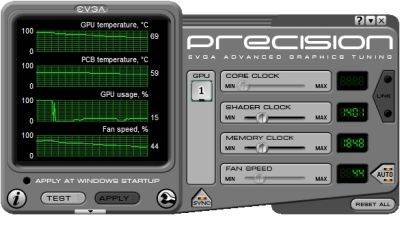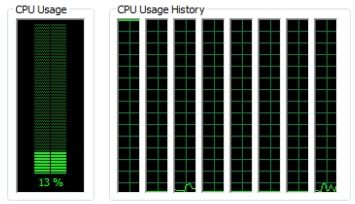# (Tested) OpenGL Geometry Instancing: GeForce GTX 480 vs Radeon HD 5870

Article Index

## 4 – Geometry Instancing Tests

Testbed:
– CPU: Intel Core i7 960 @ 3GHz
– RAM: 4GB DDR3 Corsair Dominator 1600MHz
– Mobo: Gigabyte GA-X58A-UD5
– PSU: Antec TPQ 850W
– OS: Windows 7 64-bit
– GeForce driver: R257.21

Graphics cards:
– EVGA GTX 480
– Radeon HD 5870 reference board

For each test, I read the GPU usage with EVGA Precision and the CPU usage with Windows task manager:12% or 13% means one logical CPU core is fully used.

Here are the results (default resolution: 1024×600 and default camera position):

## 20,000 instances x 18 tri/instance = 360,000 tri

– F2: FPS=43, GPU=68%, CPU=12%
– F3: FPS=55, GPU=88%, CPU=12%
F4: FPS=45, GPU=25%, CPU=12%
– F5: FPS=134, GPU=22%, CPU=12%
F6: FPS=139, GPU=24%, CPU=12%
GeForce GTX 480:
– F2: FPS=31, GPU=36%, CPU=12%
– F3: FPS=48, GPU=56%, CPU=12%
F4: FPS=117, GPU=22%, CPU=12%
– F5: FPS=150, GPU=34%, CPU=12%
F6: FPS=164, GPU=40%, CPU=12%

## 20,000 instances, 72 tri/instance = 1,440,000 tri

– F2: FPS=42, GPU=67%, CPU=12%
– F3: FPS=55, GPU=87%, CPU=12%
– F4: FPS=45, GPU=26%, CPU=12%
– F5: FPS=133, GPU=37%, CPU=12%
– F6: FPS=139, GPU=41%, CPU=12%
GeForce GTX 480:
– F2: FPS=32, GPU=21%, CPU=12%
– F3: FPS=48, GPU=33%, CPU=12%
– F4: FPS=117, GPU=22%, CPU=12%
– F5: FPS=150, GPU=37%, CPU=12%
– F6: FPS=163, GPU=44%, CPU=12%

## 20,000 instances, 450 tri/instance = 9,000,000 tri

– F2: FPS=43, GPU=69%, CPU=12%
– F3: FPS=54, GPU=87%, CPU=12%
– F4: FPS=45, GPU=64%, CPU=12%
– F5: FPS=79, GPU=99%, CPU=12%
– F6: FPS=73, GPU=99%, CPU=12%
GeForce GTX 480:
– F2: FPS=31, GPU=53%, CPU=12%
– F3: FPS=44, GPU=99%, CPU=12%
– F4: FPS=113, GPU=99%, CPU=12%
– F5: FPS=114, GPU=99%, CPU=12%
– F6: FPS=112, GPU=99%, CPU=12%

## 20,000 instances, 800 tri/instance = 16,000,000 tri

– F2: FPS=42, GPU=99%, CPU=12%
– F3: FPS=43, GPU=99%, CPU=12%
– F4: FPS=43, GPU=99%, CPU=12%
– F5: FPS=48, GPU=99%, CPU=12%
– F6: FPS=46, GPU=99%, CPU=12%
GeForce GTX 480:
– F2: FPS=31, GPU=56%, CPU=12%
– F3: FPS=42, GPU=99%, CPU=12%
– F4: FPS=66, GPU=99%, CPU=12%
– F5: FPS=67, GPU=99%, CPU=12%
– F6: FPS=66, GPU=99%, CPU=12%

## 20,000 instances, 1800 tri/instance = 36,000,000 tri

– F2: FPS=22, GPU=99%, CPU=12%
– F3: FPS=22, GPU=99%, CPU=12%
– F4: FPS=22, GPU=99%, CPU=12%
– F5: FPS=22, GPU=99%, CPU=12%
– F6: FPS=22, GPU=99%, CPU=12%
GeForce GTX 480:
– F2: FPS=30, GPU=99%, CPU=12%
– F3: FPS=30, GPU=99%, CPU=9%
– F4: FPS=31, GPU=99%, CPU=12%
– F5: FPS=32, GPU=99%, CPU=12%
– F6: FPS=32, GPU=99%, CPU=12%

## 100,000 instances, 18 tri/instance = 1,800,000 tri

– F2: FPS=9, GPU=67%, CPU=12%
– F3: FPS=12, GPU=85%, CPU=12%
– F4: FPS=10, GPU=23%, CPU=12%
– F5: FPS=33, GPU=21%, CPU=12%
F6: FPS=37, GPU=20%, CPU=12%
GeForce GTX 480:
– F2: FPS=7, GPU=34%, CPU=12%
– F3: FPS=10, GPU=54%, CPU=12%
– F4: FPS=25, GPU=14%, CPU=12%
– F5: FPS=32, GPU=25%, CPU=12%
F6: FPS=35, GPU=30%, CPU=12%

## 100,000 instances, 72 tri/instance = 7,200,000 tri

– F2: FPS=9, GPU=67%, CPU=12%
– F3: FPS=12, GPU=85%, CPU=12%
– F4: FPS=10, GPU=24%, CPU=12%
– F5: FPS=33, GPU=35%, CPU=12%
– F6: FPS=37, GPU=41%, CPU=12%
GeForce GTX 480:
– F2: FPS=7, GPU=36%, CPU=12%
– F3: FPS=10, GPU=57%, CPU=12%
– F4: FPS=25, GPU=38%, CPU=12%
– F5: FPS=32, GPU=36%, CPU=12%
– F6: FPS=35, GPU=45%, CPU=12%

## 100,000 instances, 450 tri/instance = 45,000,000 tri

– F2: FPS=9, GPU=69%, CPU=12%
– F3: FPS=12, GPU=90%, CPU=12%
– F4: FPS=10, GPU=65%, CPU=12%
– F5: FPS=17, GPU=99%, CPU=12%
– F6: FPS=16, GPU=99%, CPU=12%
GeForce GTX 480:
– F2: FPS=7, GPU=53%, CPU=12%
– F3: FPS=9, GPU=99%, CPU=12%
– F4: FPS=23, GPU=99%, CPU=12%
– F5: FPS=24, GPU=99%, CPU=10%
– F6: FPS=23, GPU=99%, CPU=8%

## 100,000 instances, 800 tri/instance = 80,000,000 tri

– F2: FPS=9, GPU=99%, CPU=12%
– F3: FPS=9, GPU=99%, CPU=12%
– F4: FPS=9, GPU=99%, CPU=12%
– F5: FPS=10, GPU=99%, CPU=12%
– F6: FPS=10, GPU=99%, CPU=12%
GeForce GTX 480:
– F2: FPS=7, GPU=56%, CPU=12%
– F3: FPS=9, GPU=99%, CPU=12%
– F4: FPS=14, GPU=99%, CPU=7%
– F5: FPS=14, GPU=99%, CPU=7%
– F6: FPS=14, GPU=99%, CPU=6%

## 100,000 instances, 1800 tri/instance = 180,000,000 tri

– F2: FPS=5, GPU=99%, CPU=8%
– F3: FPS=5, GPU=99%, CPU=8%
– F4: FPS=5, GPU=99%, CPU=8%
F5: FPS=5, GPU=99%, CPU=12%
– F6: FPS=5, GPU usage: 99%, CPU=12%
GeForce GTX 480:
– F2: FPS=6, GPU=99%, CPU=12%
– F3: FPS=6, GPU=99%, CPU=9%
– F4: FPS=7, GPU=99%, CPU=4%
F5: FPS=7, GPU=99%, CPU=3%
– F6: FPS=7, GPU=99%, CPU=4%

## 19 thoughts on “(Tested) OpenGL Geometry Instancing: GeForce GTX 480 vs Radeon HD 5870”

1.Groovounet

It would be interesting to see how the test behave with different instancing methods: instanced array, texture buffer, etc. Also 1800 triangles isn’t so much for the maximum.

2.Groovounet

Nice work anyway!

3.JeGX Post Author

Thanks Mr Groove!
I’ll update this demopack with new techniques next time (at least with instanced array). And I’ll increase the number of polygons per instance 😉

4.nicolas

FWIW, note that with more triangles, you’ll be hitting harder the triangle setup bottleneck of one triangle per clock cycle. Don’t know for the NVidia GTX 480, but this limit applies for the ATI R5xxx ; I don’t think that going beyond 180M triangles will bring you anything good for this generation of boards.

Nice summary of instanciation techniques and perfs though. Did you try to have finer grained timings with ARB_timer_query extension ?

Cheers

5.Mars_999

Yes keep these demo and tutorials coming. I enjoy reading them as it keeps me up to date on the newest features I can do with OpenGL. Plus it’s nice to see the old vs. new method of doing the same thing so once can make his own decision on what to do.

Thanks!!! keep up the good work!

6.WacKEDmaN

20,000 instances x 18 tri/instance = 360,000 tri
Geforce GTX 470@ 700 core /1800 mem
– F2: FPS=18, GPU=20%, CPU=30%
– F3: FPS=40, GPU=21%, CPU=35%
– F4: FPS=68, GPU=15%, CPU=35%
– F5: FPS=96, GPU=19%, CPU=35%
– F6: FPS=101, GPU=24%, CPU=30%

100,000 instances, 1800 tri/instance = 180,000,000 tri
Geforce GTX 470@ 700 core /1800 mem
– F2: FPS=4, GPU=67%, CPU=30%
– F3: FPS=6, GPU=99%, CPU=24%
– F4: FPS=6, GPU=99%, CPU=14%
– F5: FPS=6, GPU=99%, CPU=12%
– F6: FPS=6, GPU=99%, CPU=10%

interesting…CPU usage goes down as GPU goes up..i thought the cpu would be less stressed with the lower geometry count…

7.Matumbo

I tested with a Radeon HD 2400, Catalyst 10.6.
The F6 technique half-failed: the asteroids are there, rotating, but all shading on them is turned off (all black). But the middle planet is shaded.
I suppose this was not intended.
The driver exposes all the 3 required extensions.

8.IVXXX

20,000 instances x 18 tri/instance = 360,000 tri
ATI HD4770 @ 940 core / 4800 mem
– F2: FPS=44, GPU=50%, CPU=28%
– F3: FPS=57, GPU=58%, CPU=30%
– F4: FPS=54, GPU=40%, CPU=30%
– F5: FPS=140, GPU=42%, CPU=34%
– F6: FPS=152, GPU=34%, CPU=30% (no shadinng)

100,000 instances, 1800 tri/instance = 180,000,000 tri
ATI HD4770 @ 940 core / 4800 mem
– F2: FPS=5, GPU=99%, CPU=18%
– F3: FPS=5, GPU=99%, CPU=16%
– F4: FPS=5, GPU=99%, CPU=16%
– F5: FPS=4-8, GPU=99%, CPU=12-28%
– F6: FPS=1-6, GPU=99%, CPU=6-28% (no shadinng)

9.ca\$per

Same thing as Matumbo here. But i have Radeon 4850 on Win7 and Cat. 10.5
F6 – asteroids are all black with all triangle variations (different EXEs).

10.TopLess3D

Could you share your source code please (both C++ and GLSL), in order to learn advanced techniques and programming in OpenGL?

11.ddd

Omg, make a GRAPH, not text :p

12.krishx007

hay JeGX R u died or what?????

Not publishing any new article from 3 to 4 days???

Fight with GirlFriend?????????

13.krishx007

r u alive????????

14.codablank

where can I find the source of this demo ? or a similar source ? I get very bad performance with glDrawElements() (1000 cube instances max ) and glDrawElementsInstanced gives the same result

Yet I get a descent FPS when I run your demo (I’m on ATI 4850, opengl 2.1)

15.Ryan

Yeah, the source for this would be really handy!

16.DarkUltra

180 million eh? According to 3dmark 01, my gtx 470 does 400 million.

http://jooh.no/web/GeForce_8600_GT_vs_GTX_470_polygon_performance.png

Instancing as in the article however, does not use as much cpu power, but is also less useful than “real” cpu aware polygons.

the 470 fermi have several setup engines running in parallel instead of just one as all the previous gpus (ati and nvidia)

17.OnlyLinuxLovesUBack